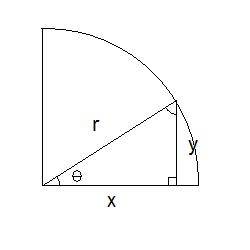# Fundamental Trigonometric Identities

Trigonometric identities are specific equalities that express one trigonometric function in terms of other trig functions. These fundamental trigonometric identities (also sometimes called basic trig identities) are fairly straightforward, but they take some work to derive them. If you are comfortable with simple derivations, you shouldn't have any problems though. Personally, I find it easier to remember the basic set of trig identities, and derive the more complex ones from those, rather than trying to memorize all of them... although some people are more comfortable just to memorize them.

These fundamental trigonometric identities are traditionally visualized as a right angle triangle inscribed within a circle of radius r, with sides formed by length x, height y, and the radius as the hypotenuse, r:The basic trig definitions and relationships can easily be observed.  I have covered these trigonometric functions in other blog posts, so I won't go into them any further here, other than to just remind you of the concept of SOHCAHTOA.  Refer to these specific posts for more information about sine, cosine, and tangent.

Sin(θ) = y/r..... opposite  /hypotenuse
Cos(θ) = x/r..... adjacent / hypotenuse
Tan(θ) = y/x..... opposite / adjacent

For the first identity that I will show you how to derive, we need to make use of the Theorem of Pythagoras (I would also here like to refer you to my post about the Theorem of Pythagoras, which itself is derived from the cosine law, for more information on this trigonometric math concept.)  If we now apply the Theorem of Pythagoras to this right angle triangle we have inscribed within a circle, we can see:

r2 = x2 + y2
Dividing everything by r2 gives:
1 = (x2)/(r2) + (y2)/(r2)
1 = (x/r)2 + (y/r)2

And then, substituting in the basic trig identities listed above, we get:

1 = [Cos(θ)]2 + [Sin(θ)]2

And that is the first of the 8 fundamental trigonometric identities. Nothing to it. In fact, this is an important mathematical expression.  As such, it has a special name or designation given to it to indicate that it is important.  It's proper name is the Pythagorean Trigonometric Identity. I'll rewrite it below in proper notation to clean it up a bit.  Notice how you can write the 2 (for the squares) in two different ways.  Either as above, like [Cos(θ)]2, where you put the trig function in brackets to square it (note that you don't square the angle!), or more commonly as below, with the 2 placed next to the trig function to indicate that it is being squared.

Similarly, we can derive another basic relationship from a standard trigonometric identity.  For this one, it starts with:
Tan(θ) = y/x

Now to substitute in some more expressions.  This one is a little more complicated than how we arrived at the Pythagorean Identity above, but follow along and you should hopefully be able to see what I do.  The next step then, is to substitute in the basic Sine and Cosine definitions from the list at the top of this page (isolated for x and y, respectively) to give:

Tan(θ) = (r x Sin(θ)) / (r x Cos(θ))
Tan(θ) = Sin(θ) / Cos(θ)

And that's it again. Like the Pythagorean Trigonometric Identity I described above, this one also has a specific name.  This time, this one is called the Ratio Identity:Those are now two of the simplest basic trig identities from which most of the others can be derived.  As I mentioned at the top, I always find that it is easier to memorize HOW to derive these fundamental trigonometric identities, rather than memorizing them explicitly and then possibly getting confused.  You WILL memorize them eventually (which always happens when you work with them so much!), but when you are first trying to understand them, I believe that it is always better to understand what is going on instead of just memorizing the final solutions.  This approach is a very wise strategy to apply throughout your mathematics training!

*Update 03-April-2012:  I have recently come back to this topic of basic trig identities, and have started a completely new post called the Trig Identities Cheat Sheet.  Please visit that site for a more thorough explanation of some of these derivations and fundamental identities of trigonometric functions.  Also, please click the +1 button below if you found this helpful!  Thanks.